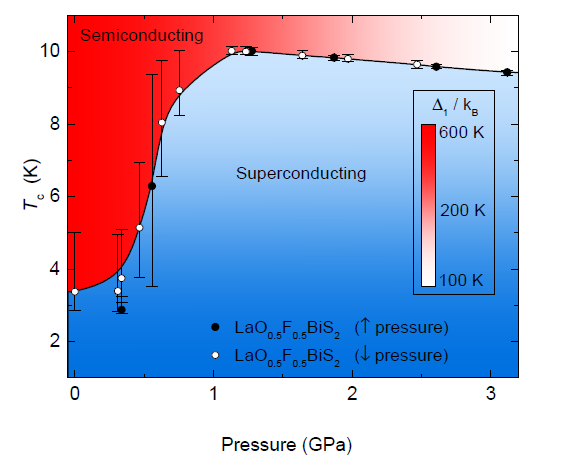Skip Navigation Links.

### Tc vs. P Phase Diagram for the Superconducting Compound LaO0.5F0.5BiS2Description:

This figure represents the phase diagram for the superconducting compound LaO0.5F0.5BiS2 in which the superconducting transition temperature Tc (in degrees Kelvin) is plotted as a function of applied pressure P (in units of GigaPascal or GPa). Tc was determined from electrical resistivity measurements (not shown) where the superconducting transition is indicated by a precipitous drop in electrical resistivity after which the compound exhibits zero electrical resistance in the superconducting state. The basic components of this graph include: a data set (filled symbols) representing Tc obtained during electrical resistivity measurements upon an increase in pressure; a data set (open symbols) representing Tc obtained during electrical resistivity measurements upon unloading pressure; a b-spline curve (black curve through data sets) as a guide to the eye for the evolution of Tc with pressure; the b-spline curve separates the graph into two color schemes each representing a different phase of the compound for a given temperature and pressure. The region corresponding to the semiconducting phase is represented by a horizontal gradient from red to white while the region corresponding to the superconducting phase is represented by softer vertical gradient in blue. The color gradient from red to white in the semiconducting region represents the suppression of the semiconducting behavior with increasing pressure which was manifested by a decreasing energy gap Δ1. The values of the energy gap Δ1 (in units of Kelvin) are indicated in the vertical rectangular false-color legend containing the same red to white color gradient as in the semiconducting region. From the graph, one should be able to determine the phase of the compound for a given temperature and pressure as well as the approximate value of the energy gap Δ1 for the case of the semiconducting phase.

The evolution of Tc in the phase diagram is characterized by the filled and open symbols, i.e., the data, along with a b-spline line as a guide to the eye. The vertical bars with caps represent the width of the superconducting transition as determined from the electrical resistivity data (not shown). The rapid increase in Tc over the small window of pressure from 0.3 to 1.0 GPa indicates the existence of a pressure-induced transition between a superconducting phase with Tc ~ 3.1 K to a superconducting phase with a higher Tc ~10 K. The evolution of Tc is shown to be reversible with pressure as indicated from the filled (open) symbols which denote measurements of Tc that were performed upon increasing (decreasing) pressure. These two data sets were plotted from separate columns allowing for the contrasting filled and open designations.

The construction of the plot is best described as a three layer plot. The bottom layer consists of the axes, data and the red to white color gradient. The middle layer consists of the b-spline line fitted through both sets of data and the blue semiconducting region chosen as a fill option for the region below the b-spline curve. The top layer consists of the false-color legend in the form of a vertical rectangle with the same red to white gradient used in the bottom layer of the plot. The rectangle was constructed from a “dummy” data set such that when plotted with the “line and symbol” option the plotted curve formed the shape of a rectangle. The space within the rectangular data set was filled with the same gradient options much the same way as the other two layers were constructed.

This graph was contributed by Christian Wolowiec, a PhD Physics graduate student from the Maple Group Lab at the University of California, San Diego Physics Department.

This graph is published in:
C. T. Wolowiec, D. Yazici, B. D. White, K. Huang, and M. B. Maple, Pressure-induced enhancement of superconductivity and suppression of semiconducting behavior in LnO0.5F0.5BiS2 (Ln=La,Ce) compounds, Phys. Rev. B 88, 064503 (2013)